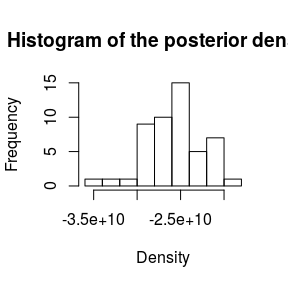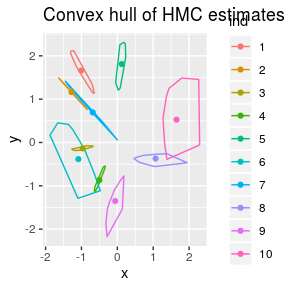# DrBats Model Fit

## Overview

This document is part of the “DrBats” project whose goal is to implement exploratory statistical analysis on large sets of data with uncertainty. The idea is to visualize the results of the analysis in a way that explicitely illustrates the uncertainty in the data.

The “DrBats” project applies a Bayesian Latent Factor Model.

This project involves the following persons, listed in alphabetical order :

• Bénédicte Fontez (aut)

• Susan Holmes (aut)

• Gabrielle Weinrott (cre, aut)

## Fitting the model to simulated data

require(DrBats)
mycol<-c("#ee204d", "#1f75fe", "#1cac78", "#ff7538", "#b4674d", "#926eae",
"#fce883", "#000000", "#78dbe2", "#6e5160", "#ff43a4")

To fit the model, you need to specify a bunch of parameters: the type of model (“PLT” or “sparse”), the family of variance priors (“IG” or “cauchy” for now), the program you want to use (only “stan” is implemented for the time being), a dataset projected onto a histogram basis, the number of latent factors (“D”), and then some other habitual MCMC things (iterations, thinning, burnin).

Then you can execute the modelFit() function with the chosen parameters. STAN calculations can be long, so the following example is intentionally very small, with few iterations and few chains on the toy dataset.

If you want to estimate many parameteres and require a large number of iterations, you may want to consider running the following commands on a server or a desktop machine.

data("toydata")
proj.data <- toydata$Y.simul$Y
stanfit <- modelFit(model = "PLT", var.prior = "IG", prog = "stan", parallel = T, Xhisto = proj.data,
nchains = 2, nthin = 10, niter = 1000, R = toydata$wlu$Q)

First we must convert the stanfit object to an mcmc.list. In this step we have loaded a stanfit object that is available in the package (with more iterations and chains than in the example above). We can do this using the function coda.obj().

data("stanfit")
coda.plt <- coda.obj(stanfit)

We can plot the posterior density of the likelihood:

post <- postdens(coda.plt, Y = toydata$Y.simul$Y, D = toydata$wlu$D, chain = 1)
hist(post, main = "Histogram of the posterior density", xlab = "Density")We can also plot the individual projections on the plane spanned by the estimated latent factors, with $$90 \%$$ uncertainty regions.

beta.res <- visbeta(coda.plt, toydata$Y.simul$Y, toydata$wlu$D, chain = 1, axes = c(1, 2), quant = c(0.05, 0.95))

ggplot2::ggplot() +
ggplot2::geom_path(data = beta.res$contour.df, ggplot2::aes(x = x, y = y, colour = ind)) + ggplot2::geom_point(data = beta.res$mean.df, ggplot2::aes(x = x, y = y, colour = ind)) +
ggplot2::ggtitle("Convex hull of HMC estimates of the scores")